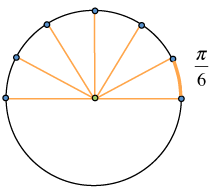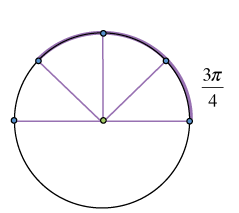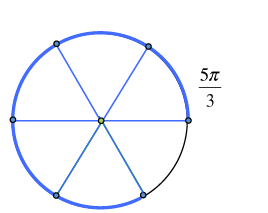### Home > PC > Chapter 2 > Lesson 2.3.6 > Problem2-151

2-151.

Sketch a graph of the unit circle with the angles specified below.

1. $\frac { \pi } { 6 }$

Divide the top half of the circle ($\pi$ radians) into six pieces. Select the arc of the first piece.1. $\frac{3\pi}{4}$

Divide the top half of the circle ($\pi$ radians) into four pieces. Select the arc of three of these pieces.1. $\frac{5\pi}{3}$

Divide the top and bottom half of the circle into three pieces each. Select the arc of five of these pieces.